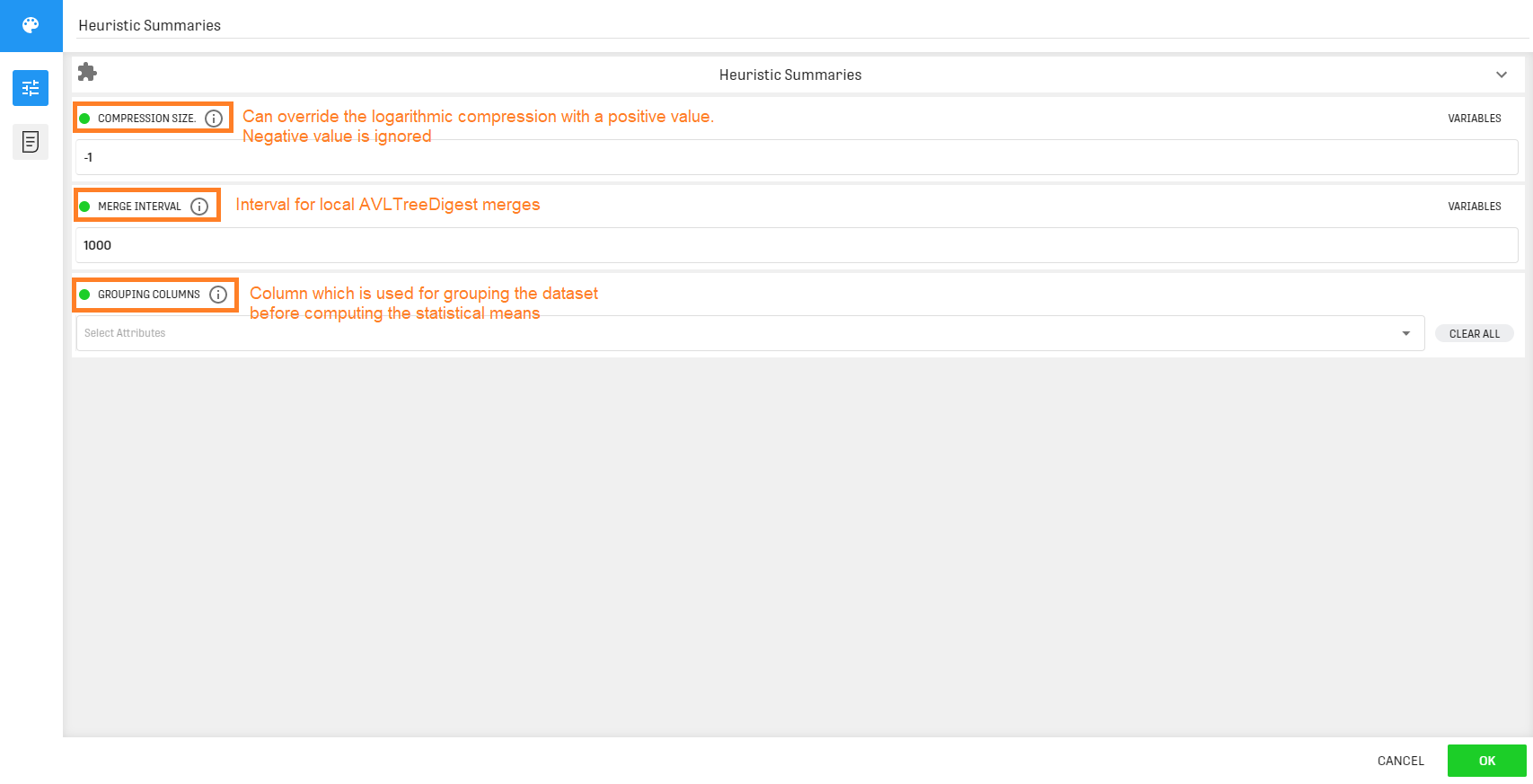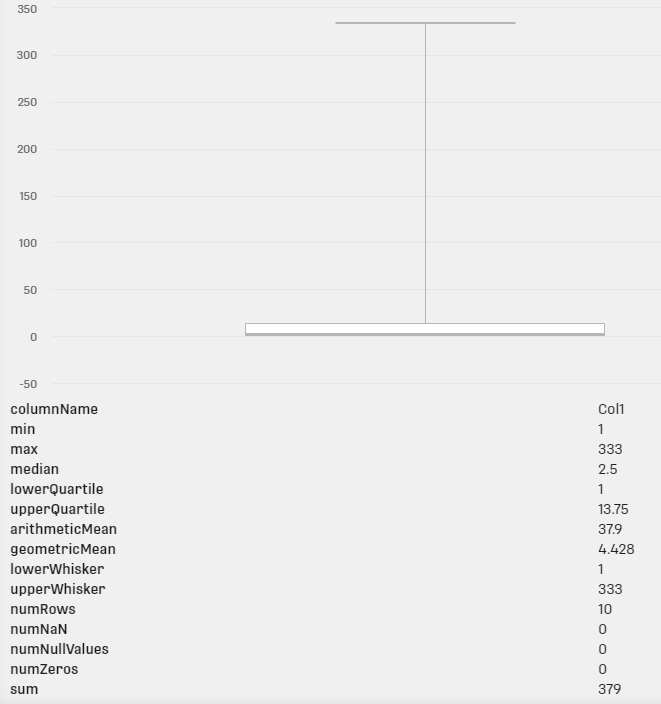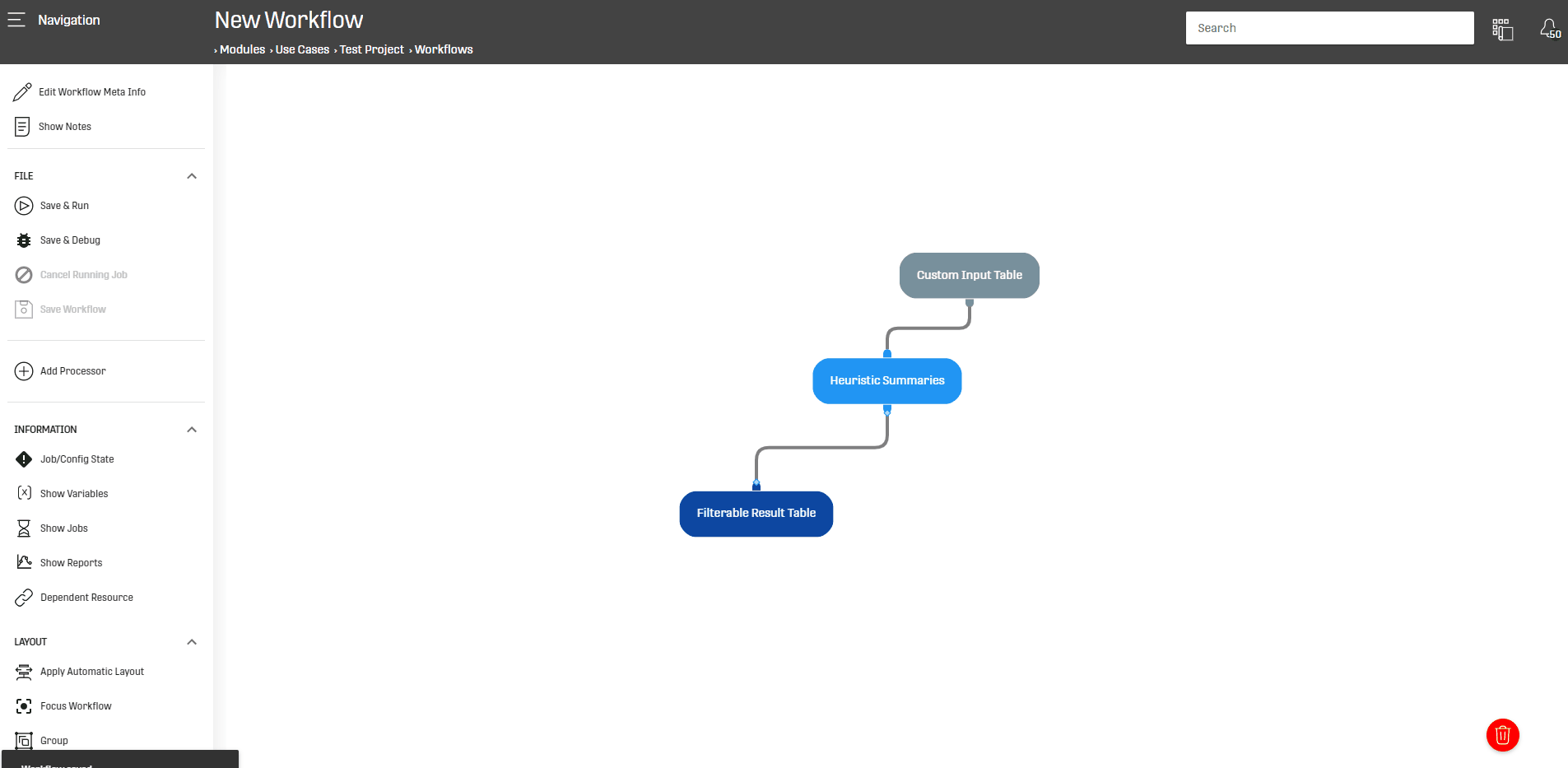# Heuristic Summaries Processor

Modified on Tue, 30 Nov 2021 at 11:39 AM

# Overview

This processor calculates certain statistical measures (exp: Mean, Median ...) and visualizes the Data in boxplot.

# Motivation

To gain important insights from Data and extract helpful information embedded in it, it is necessary to understand this Data which this can be achieved by applying some Descriptive Analysis on the Data of interest.

The Heuristic Summaries Processor helps generate significant statistics from the input Data.

# Configuration

The processor requires a Dataset containing at least one numeric/ratio scaled column/variable.

This processor is generally linked to a Load Processor to interpret input Data, or maybe after applying some transformations on this Data.

The configuration menu of the processor is the following:Compression Size: the higher the value, the higher precision will be BUT execution time rises and memory consumption will be high.

Merge Interval: define interval for merging tree centroids

NOTE THAT:
• these two configuration fields are experimental
• the processor does not infer column type meaning that if a column is declared as of type "String" but contains only numbers the processor will NOT take it into account

# Output

This processor provides two outputs:

• within the processor: Displays a Boxplot graph accompanied with multiple statistical measures (min, max, median ...) for each numeric Column from the input Dataset# Example

In this example the Heuristic Summary Processor will be applied on a simple Dataset to extract statistical measures from this Data:# Related Articles

Distinct Summary

Distinct Textual Summary